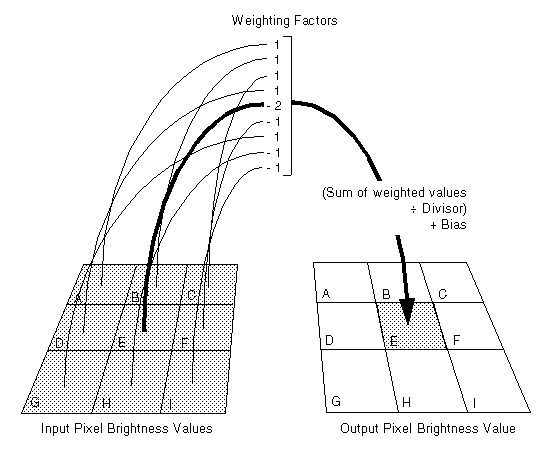﻿ SpatialFilterCommand Constructor(int,int,int[]) (SpatialFilterCommand) | Leadtools.ImageProcessing.Effects | Raster, Medical, Document Help
←Select platform
In This Topic ▼

# SpatialFilterCommand Constructor(int,int,int[])

Summary
Initializes a new SpatialFilterCommand class object with explicit parameters.
Syntax
C#
VB
Objective-C
C++
Java
````public SpatialFilterCommand( `
`   int divisor, `
`   int bias, `
`   int[] matrix `
`) ````
````Public Function New( _ `
`   ByVal divisor As Integer, _ `
`   ByVal bias As Integer, _ `
`   ByVal matrix() As Integer _ `
`) ````
````- (instancetype)initWithDivisor:(NSInteger)divisor `
`                           bias:(NSInteger)bias `
`                         matrix:(nullable const int *)matrix `
`                   matrixLength:(NSUInteger)matrixLength ````
````public SpatialFilterCommand( `
`   int divisor,  `
`   int bias,  `
`   int[] matrix `
`) ````
````public: `
`SpatialFilterCommand(  `
`   int divisor, `
`   int bias, `
`   array<int>^ matrix `
`) ````

#### Parameters

divisor
Number used to divide the sum of weighted values.

bias
Number added to the weighted average.

matrix
The array of weighting factors. The factors apply to pixels in the neighborhood read left to right and top to bottom, as shown below:Example

Run the SpatialFilterCommand on an image.

C#
VB
````using Leadtools; `
`using Leadtools.Codecs; `
`using Leadtools.ImageProcessing.Effects; `
` `
`public void SpatialFilterConstructorExample_S2() `
`{ `
`   // Load an image `
`   RasterCodecs codecs = new RasterCodecs(); `
`   codecs.ThrowExceptionsOnInvalidImages = true; `
` `
`   RasterImage image = codecs.Load(Path.Combine(LEAD_VARS.ImagesDir, "ImageProcessingDemo\\NaturalFruits.jpg")); `
` `
`   // Prepare the command `
`   SpatialFilterCommand command1 = new SpatialFilterCommand(SpatialFilterCommandPredefined.EmbossSouth); `
`   int[] data = command1.Matrix; `
`   SpatialFilterCommand command = new SpatialFilterCommand(command1.Divisor, command1.Bias, data); `
`   //Apply the filter. `
`   command.Run(image); `
` `
`} `
` `
`static class LEAD_VARS `
`{ `
`   public const string ImagesDir = @"C:\LEADTOOLS21\Resources\Images"; `
`} ````
````Imports Leadtools `
`Imports Leadtools.Codecs `
`Imports Leadtools.ImageProcessing.Effects `
` `
`Public Sub SpatialFilterConstructorExample_S2() `
`   Dim codecs As New RasterCodecs() `
`   codecs.ThrowExceptionsOnInvalidImages = True `
` `
`   Dim leadImage As RasterImage = codecs.Load(Path.Combine(LEAD_VARS.ImagesDir, "ImageProcessingDemo\\NaturalFruits.jpg")) `
` `
`   ' Prepare the command `
`   Dim command1 As SpatialFilterCommand = New SpatialFilterCommand(SpatialFilterCommandPredefined.EmbossSouth) `
`   Dim data() As Integer = command1.Matrix `
`   Dim command As SpatialFilterCommand = New SpatialFilterCommand(command1.Divisor, command1.Bias, data) `
`   'Apply the filter. `
`   command.Run(leadImage) `
`   codecs.Save(leadImage, Path.Combine(LEAD_VARS.ImagesDir, "Result.jpg"), RasterImageFormat.Jpeg, 24) `
` `
`End Sub `
` `
`Public NotInheritable Class LEAD_VARS `
`   Public Const ImagesDir As String = "C:\LEADTOOLS21\Resources\Images" `
`End Class ````
Requirements